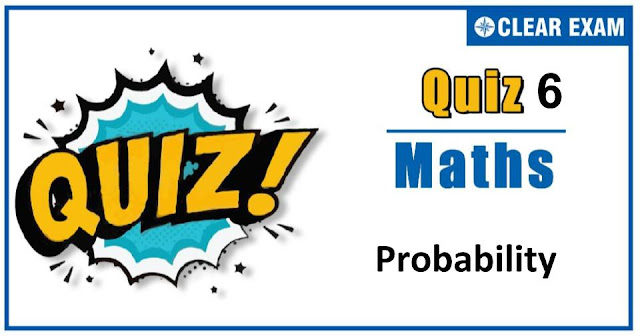## [LATEST]\$type=sticky\$show=home\$rm=0\$va=0\$count=4\$va=0

As per analysis for previous years, it has been observed that students preparing for JEE MAINS find Mathematics out of all the sections to be complex to handle and the majority of them are not able to comprehend the reason behind it. This problem arises especially because these aspirants appearing for the examination are more inclined to have a keen interest in Mathematics due to their ENGINEERING background.

Furthermore, sections such as Mathematics are dominantly based on theories, laws, numerical in comparison to a section of Engineering which is more of fact-based, Physics, and includes substantial explanations. By using the table given below, you easily and directly access to the topics and respective links of MCQs. Moreover, to make learning smooth and efficient, all the questions come with their supportive solutions to make utilization of time even more productive. Students will be covered for all their studies as the topics are available from basics to even the most advanced.

Q1. A parents has two children. If one of them is boy, then the probability that other is, also a boy, is
•  1/2
•  1/4
•  1/3
•  None of these
Solution
(c) Total cases are BB.BG,GB,GG=4 Favourable cases are BB,BG,GB=3 Let P(A)=Probability of a boy in two children =3/4 Let P(B)=The probability that the second child is also a boy =1/4 Here, P(A∩B)=1/4 ∴P(B/A)=(P(A∩B))/(P(A))=(1/4)/(3/4)=1/3

Q2.If the probability for A to fail in an examination is 0.2 and that for B is 0.3, then the probability that either A or B fails, is
•  0.38
•  0.44
•  0.50
•  0.94
Solution
(b) P(Ac)=0.2,P(Bc)=0.3 P(A)=0.8,P(B)=0.7 ∴ Required probability =P(Ac)P(B)+ P(A) P(Bc)+P(Ac)P(Bc) =0.2×0.7+0.8×0.3+0.2×0.3 =0.44
Q3.  Two dice are thrown together. Then, the probability that the sum of numbers appearing on them is a prime number, is
•   5/12
•  7/18
•  13/36
•  11/36
Solution
(a) The prime numbers between 2 to12 are 2, 3, 5, 7, 11 Case I When sum is 2, total cases are (1, 1) ie,1 Case II When sum is 3, total cases are (1, 2), (2, 1) ie,2 Case III When sum is 5, total cases are (1, 4), (2, 3), (4, 1), (3, 2) ie,4 Case IV When sum is 7, total cases are (1, 6), (2, 5), (3, 4), (6, 1), (5, 2), (4, 3) ie,6 Case V When sum is 11, total cases are (5, 6), (6, 5) ie,2 ∴ Required probability=15/36=5/12

Q4. Three of the six vertices of a regular hexagon are chosen at random. The probability that the triangle with these three vertices is equilateral, equals
•  1/2
•  1/5
•  1/10
•  1/20
Solution
(c) We can choose three vertices out of 6 in 6 C3=20 ways. Chosen vertices can form an equilateral triangle in just two ways viz,A1,A3,A5 and A2,A4,A6. ∴ Required probability =2/20=1/10.

Q5.If X is a binomial variate with the range {0,1,2,3,4,5,6} and P(X=2)=4P(X=4), then the parameter p of X is
•  1/3
•  1/2
•  2/3
•  3/4
Solution
(a) Here, n=6 According to the question 6 C2 p2 q4=4.6 C4 p4 q2 ⇒ q2=4p2 ⇒ (1-p)2=4p2 ⇒ 3p2+2P-1=0 ⇒ (p+1)(3p-1)=0 ⇒p=1/3(∵p cannot be negative)

Q6. Let A and B are two events and P(A' )=0.3,P(B)=0.4,P(A∩B' )=0.5, thenP(A∪B') is
•  0.5
•  0.8
• 1
•  0.1
Solution
(b) Here, P(B' )=1-0.4=0.6 and P(A)=1-0.3=0.7 ∴ P(A∪B' )=P(A)+P(B' )-P(A∩B') =0.7+0.6-0.5=0.8

Q7.If E and F are events with P(E)≤P(F) and P(E∪F)>0, then
•  Occurrence of E⇒occurence of F
•  Occurrence of F⇒occurence of E
•  Non-occurrence of E⇒Non-ccurence of F
•  None of the above
Solution
(d) Explanation not available

Q8.The probability that A speaks truth is 4/5 while this probability for B is 3/4. The probability that they contradict each other when asked to speak on a fact, is
•  3/20
•  1/5
•  7/20
•  4/5
Solution
(c) Given probability of speaking truth are P(A)=4/5 and P(B)=3/4 And their corresponds probabilities of not speaking truth are P(Ac)=1/5 and P(Bc )=1/4 The probability that they contradict each other =P(A)×P(Bc )+P(Ac)×P(B) =4/5×1/4+1/5×3/4 =1/5+3/20 =7/20

Q9.A biased die is tossed and the respective probabilities for various faces to turn up are Face : 1 2 3 4 5 6 Probability : 0.1 0.24 0.19 0.18 0.15 0.14 If an even face has turned up, then the probability that it is face 2 or face 4, is
•  0.25
•  0.42
•  0.75
•  0.9
Solution
(c) Let A be the even that an even face turns up and B be the event that it is 2 or 4. Then, P(A)=0.24+0.18+0.14=0.56 and, P(B)=0.24+0.18=0.42 ∴ Required probability =P(B/A) ⇒ Required probability =(P(A∩B))/(P(A))=(P(B))/(P(A)) [∵B⊂A] ⇒ Required probability =0.42/0.56=3/4=0.75

Q10. The probability that the same number appear on throwing three dice simultaneously, is
•   1/6
•  1/36
•  5/36
• None of these
Solution
(b) Total number of ways =6×6×6 Favourable number of ways = 6 ∴ Required probability =6/(6×6×6)=1/36## Want to know more

Want to Know More
Please fill in the details below:

## Latest NEET Articles\$type=three\$c=3\$author=hide\$comment=hide\$rm=hide\$date=hide\$snippet=hide

Name

ltr
item
Best IIT JEE Coaching Institute in Delhi | NEET Coaching Institute in Delhi: Probability-quiz-6
Probability-quiz-6
https://1.bp.blogspot.com/-rzDLgchtT-c/X41JBiaSAjI/AAAAAAAAEb8/xq4EyBcA-RQqciscX1DHl9Y7CmS35B4XQCLcBGAsYHQ/w640-h336/Quiz%2BImage%2BTemplate%2B%252845%2529.jpg
https://1.bp.blogspot.com/-rzDLgchtT-c/X41JBiaSAjI/AAAAAAAAEb8/xq4EyBcA-RQqciscX1DHl9Y7CmS35B4XQCLcBGAsYHQ/s72-w640-c-h336/Quiz%2BImage%2BTemplate%2B%252845%2529.jpg
Best IIT JEE Coaching Institute in Delhi | NEET Coaching Institute in Delhi
https://www.cleariitmedical.com/2020/10/probability-quiz-6.html
https://www.cleariitmedical.com/
https://www.cleariitmedical.com/
https://www.cleariitmedical.com/2020/10/probability-quiz-6.html
true
7783647550433378923
UTF-8